Cloudeeeee

4035zlbcarderIceCola丶Lucky_1002skimjinycIERkarmenkoacwwgynfksdlvWiselnnKkkkkkkkkKK

Cloudeeeee
14小时前

1. 题目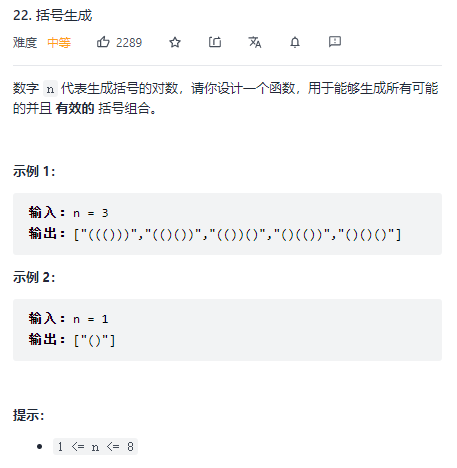2. 读题（需要重点注意的东西）

① 任意前缀中，左括号数量 >= 右括号数量
② 左右括号数量相等

① 填(的情况：只要还有左括号，就能填左括号
② 填)的情况：还有右括号左括号数量 > 右括号数量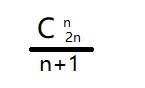3. 解法

---------------------------------------------------解法---------------------------------------------------

class Solution {
List<String> list = new ArrayList<>();
public List<String> generateParenthesis(int n) {
// dfs(括号对数，当前已经使用的左括号，当前已经使用的右括号，当前的括号序列)
dfs(n,0,0,"");
return list;
}
private void dfs(int n,int lc,int rc,String cur){
if(lc == n && rc == n) list.add(cur);
else{
if(lc <= n) dfs(n,lc+1,rc,cur+'(');
if(rc <= n && lc > rc) dfs(n,lc,rc+1,cur+')');
}
}
}

• 递归
• dfs

Cloudeeeee
14小时前

1. 题目2. 读题（需要重点注意的东西）

① 任意前缀中，左括号数量 >= 右括号数量
② 左右括号数量相等

① 填(的情况：只要还有左括号，就能填左括号
② 填)的情况：还有右括号左括号数量 > 右括号数量3. 解法

---------------------------------------------------解法---------------------------------------------------

class Solution {
List<String> list = new ArrayList<>();
public List<String> generateParenthesis(int n) {
// dfs(括号对数，当前已经使用的左括号，当前已经使用的右括号，当前的括号序列)
dfs(n,0,0,"");
return list;
}
private void dfs(int n,int lc,int rc,String cur){
if(lc == n && rc == n) list.add(cur);
else{
if(lc <= n) dfs(n,lc+1,rc,cur+'(');
if(rc <= n && lc > rc) dfs(n,lc,rc+1,cur+')');
}
}
}

• 递归
• dfs

Cloudeeeee
22小时前

1. 题目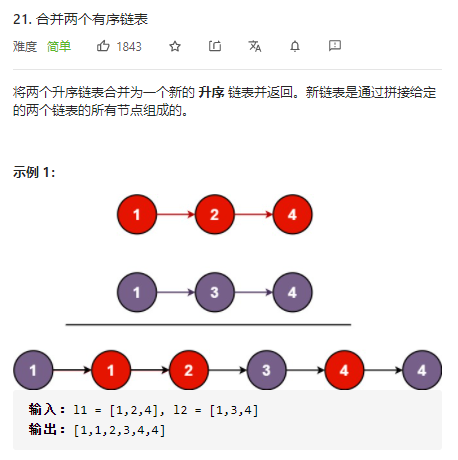2. 读题（需要重点注意的东西）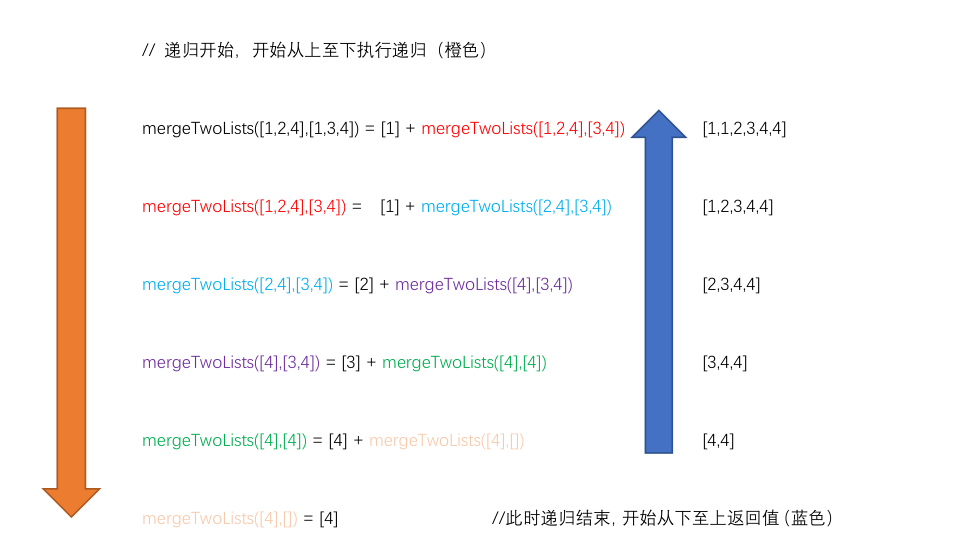3. 解法

/**
* public class ListNode {
*     int val;
*     ListNode next;
*     ListNode() {}
*     ListNode(int val) { this.val = val; }
*     ListNode(int val, ListNode next) { this.val = val; this.next = next; }
* }
*/
class Solution {
public ListNode mergeTwoLists(ListNode l1, ListNode l2) {
if(l1 == null || l2 == null) return l1 == null ? l2 : l1;
ListNode tail = new ListNode(0);
ListNode cur = tail;
while(l1 != null && l2 != null){
if(l1.val < l2.val){
cur.next = l1;
l1 = l1.next;
}
else{
cur.next = l2;
l2 = l2.next;
}
cur = cur.next;
}
cur.next = l1 == null ? l2 : l1;
return tail.next;
}
}

/**
* public class ListNode {
*     int val;
*     ListNode next;
*     ListNode() {}
*     ListNode(int val) { this.val = val; }
*     ListNode(int val, ListNode next) { this.val = val; this.next = next; }
* }
*/
class Solution {
public ListNode mergeTwoLists(ListNode l1, ListNode l2) {
if(l1 == null || l2 == null) return l1 == null ? l2 : l1;
if(l1.val < l2.val){
l1.next = mergeTwoLists(l1.next,l2);
return l1;
}
else{
l2.next = mergeTwoLists(l1,l2.next);
return l2;
}
}
}

Cloudeeeee
22小时前

1. 题目2. 读题（需要重点注意的东西）3. 解法

/**
* public class ListNode {
*     int val;
*     ListNode next;
*     ListNode() {}
*     ListNode(int val) { this.val = val; }
*     ListNode(int val, ListNode next) { this.val = val; this.next = next; }
* }
*/
class Solution {
public ListNode mergeTwoLists(ListNode l1, ListNode l2) {
if(l1 == null || l2 == null) return l1 == null ? l2 : l1;
ListNode tail = new ListNode(0);
ListNode cur = tail;
while(l1 != null && l2 != null){
if(l1.val < l2.val){
cur.next = l1;
l1 = l1.next;
}
else{
cur.next = l2;
l2 = l2.next;
}
cur = cur.next;
}
cur.next = l1 == null ? l2 : l1;
return tail.next;
}
}

/**
* public class ListNode {
*     int val;
*     ListNode next;
*     ListNode() {}
*     ListNode(int val) { this.val = val; }
*     ListNode(int val, ListNode next) { this.val = val; this.next = next; }
* }
*/
class Solution {
public ListNode mergeTwoLists(ListNode l1, ListNode l2) {
if(l1 == null || l2 == null) return l1 == null ? l2 : l1;
if(l1.val < l2.val){
l1.next = mergeTwoLists(l1.next,l2);
return l1;
}
else{
l2.next = mergeTwoLists(l1,l2.next);
return l2;
}
}
}

Cloudeeeee
23小时前

1. 题目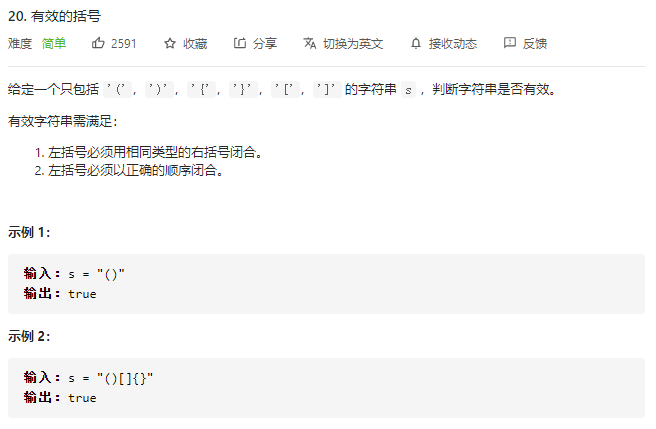2. 读题（需要重点注意的东西）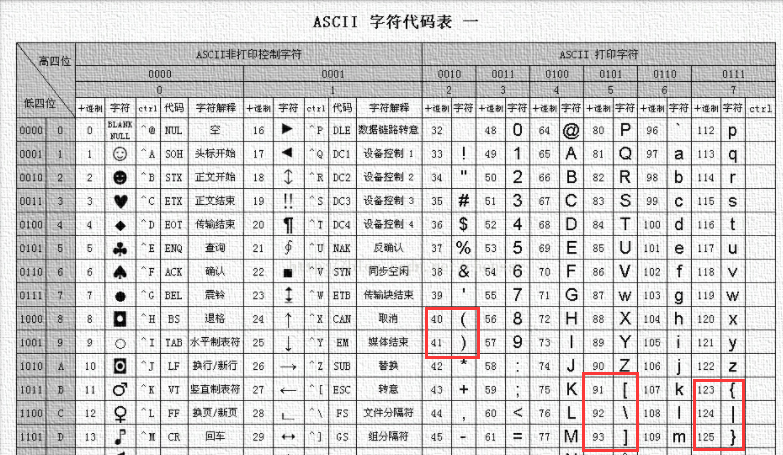3. 解法

------------------------------------解法1：优化前----------------------------------------------

class Solution {
public boolean isValid(String s) {
Stack<Character> stack = new Stack<>();
int len = s.length();
int i = 0;
while(len > 0){
// 遇到左括号 ( [ } 进栈
if(s.charAt(i) == '(' || s.charAt(i) == '[' || s.charAt(i) == '{') stack.push(s.charAt(i));
// 遇到右括号 ) ] } 出栈配对
if(s.charAt(i) == ')'){
if(stack.isEmpty()) return false;
char c = stack.pop();
if(c != '(') return false;
}
if(s.charAt(i) == ']'){
if(stack.isEmpty()) return false;
char c = stack.pop();
if(c != '[') return false;
}
if(s.charAt(i) == '}'){
if(stack.isEmpty()) return false;
char c = stack.pop();
if(c != '{') return false;
}
i ++ ;
len --;
}
return stack.isEmpty();
}
}

-------------------------------解法2：通过ASCII码进行优化后-----------------------------

class Solution {
public boolean isValid(String s) {
Stack<Character> stack = new Stack<>();
int len = s.length();
int i = 0;
for(char c : s.toCharArray()){
// 遇到左括号 ( [ } 进栈
if(c == '(' || c == '[' || c == '{') stack.push(c);
// 遇到右括号 ) ] } 出栈配对
else{
if(stack.isEmpty() == false && Math.abs(c-stack.peek()) <= 2) stack.pop();
else return false;
}
}
return stack.isEmpty();
}
}

Cloudeeeee
23小时前

1. 题目2. 读题（需要重点注意的东西）3. 解法

------------------------------------解法1：优化前----------------------------------------------

class Solution {
public boolean isValid(String s) {
Stack<Character> stack = new Stack<>();
int len = s.length();
int i = 0;
while(len > 0){
// 遇到左括号 ( [ } 进栈
if(s.charAt(i) == '(' || s.charAt(i) == '[' || s.charAt(i) == '{') stack.push(s.charAt(i));
// 遇到右括号 ) ] } 出栈配对
if(s.charAt(i) == ')'){
if(stack.isEmpty()) return false;
char c = stack.pop();
if(c != '(') return false;
}
if(s.charAt(i) == ']'){
if(stack.isEmpty()) return false;
char c = stack.pop();
if(c != '[') return false;
}
if(s.charAt(i) == '}'){
if(stack.isEmpty()) return false;
char c = stack.pop();
if(c != '{') return false;
}
i ++ ;
len --;
}
return stack.isEmpty();
}
}

-------------------------------解法2：通过ASCII码进行优化后-----------------------------

class Solution {
public boolean isValid(String s) {
Stack<Character> stack = new Stack<>();
int len = s.length();
int i = 0;
for(char c : s.toCharArray()){
// 遇到左括号 ( [ } 进栈
if(c == '(' || c == '[' || c == '{') stack.push(c);
// 遇到右括号 ) ] } 出栈配对
else{
if(stack.isEmpty() == false && Math.abs(c-stack.peek()) <= 2) stack.pop();
else return false;
}
}
return stack.isEmpty();
}
}

Cloudeeeee
1天前

1. 题目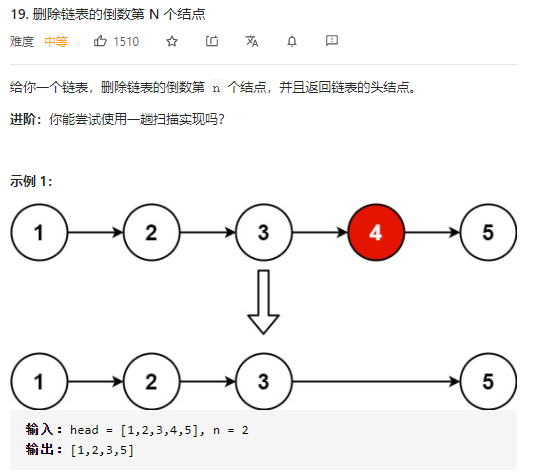3. 解法

/**
* public class ListNode {
*     int val;
*     ListNode next;
*     ListNode() {}
*     ListNode(int val) { this.val = val; }
*     ListNode(int val, ListNode next) { this.val = val; this.next = next; }
* }
*/
class Solution {
public ListNode removeNthFromEnd(ListNode head, int n) {
// 快指针先走n步
while (n > 0) {
fast = fast.next;
n--;
}
// 表明要删除的是第一个节点
if (fast == null) return head.next;
// 慢指针和快指针一起走
while (fast.next != null) {
fast = fast.next;
slow = slow.next;
}
// 当快指针走到终点时，慢指针的下一个节点就是需要删除的节点
slow.next = slow.next.next;
}
}

Cloudeeeee
1天前

1. 题目3. 解法

/**
* public class ListNode {
*     int val;
*     ListNode next;
*     ListNode() {}
*     ListNode(int val) { this.val = val; }
*     ListNode(int val, ListNode next) { this.val = val; this.next = next; }
* }
*/
class Solution {
public ListNode removeNthFromEnd(ListNode head, int n) {
// 快指针先走n步
while (n > 0) {
fast = fast.next;
n--;
}
// 表明要删除的是第一个节点
if (fast == null) return head.next;
// 慢指针和快指针一起走
while (fast.next != null) {
fast = fast.next;
slow = slow.next;
}
// 当快指针走到终点时，慢指针的下一个节点就是需要删除的节点
slow.next = slow.next.next;
}
}

Cloudeeeee
1天前

1. 题目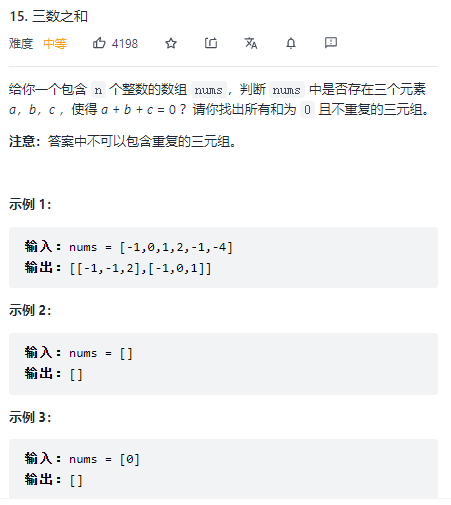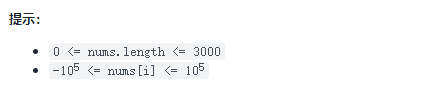2. 读题（需要重点注意的东西）

[LeetCode] 15. 三数之和（java实现）完全相同。

3. 解法

---------------------------------------------------解法---------------------------------------------------

class Solution {
public List<List<Integer>> fourSum(int[] nums, int target) {
List<List<Integer>> list = new ArrayList<>();
if(nums.length == 0) return new ArrayList<>();
int n = nums.length;
Arrays.sort(nums);
// 定下nums[i]
for(int i = 0;i < n;i++){
// 去重
if(i != 0 && nums[i] == nums[i - 1]) continue;
// 定下nums[j]
for(int j = i + 1;j < n - 2;j++){
// 去重
if(j != i+1 && nums[j] == nums[j-1]) continue;
int k = j + 1,m = n-1;
// 双指针
while(k < m){
int sum = nums[i] + nums[j] + nums[k] + nums[m];
if(sum > target){
m--;
continue;
}
if(sum < target){
k++;
continue;
}else if(sum == target){
}
// 去重
do{k++;}while(k < m && nums[k] == nums[k - 1]);
do{m--;}while(k < m && nums[m] == nums[m + 1]);
}
}
}
return list;
}
}

Cloudeeeee
1天前

1. 题目2. 读题（需要重点注意的东西）

[LeetCode] 15. 三数之和（java实现）完全相同。

3. 解法

---------------------------------------------------解法---------------------------------------------------

class Solution {
public List<List<Integer>> fourSum(int[] nums, int target) {
List<List<Integer>> list = new ArrayList<>();
if(nums.length == 0) return new ArrayList<>();
int n = nums.length;
Arrays.sort(nums);
// 定下nums[i]
for(int i = 0;i < n;i++){
// 去重
if(i != 0 && nums[i] == nums[i - 1]) continue;
// 定下nums[j]
for(int j = i + 1;j < n - 2;j++){
// 去重
if(j != i+1 && nums[j] == nums[j-1]) continue;
int k = j + 1,m = n-1;
// 双指针
while(k < m){
int sum = nums[i] + nums[j] + nums[k] + nums[m];
if(sum > target){
m--;
continue;
}
if(sum < target){
k++;
continue;
}else if(sum == target){
}
// 去重
do{k++;}while(k < m && nums[k] == nums[k - 1]);
do{m--;}while(k < m && nums[m] == nums[m + 1]);
}
}
}
return list;
}
}